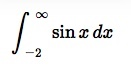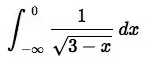# Improper Integrals: Simple Definition, Examples

## What is an Improper Integral?

Watch the video for an overview:

An improper integral is a definite integral—one with upper and lower limits—that goes to infinity in one direction or another. Either one of its limits are infinity, or the integrand (that function inside the interval, usually represented by f(x)) goes to infinity in the integral.

Improper integrals are integrals you can’t immediately solve because of the infinite limit(s) or vertical asymptote in the interval. The reason you can’t solve these integrals without first turning them into a proper integral (i.e. one without infinity) is that in order to integrate, you need to know the interval length. And if your interval length is infinity, there’s no way to determine that interval. The workaround is to turn the improper integral into a proper one and then integrate by turning the integral into a limit problem.

Limits for improper integrals do not always exist; An improper integral is said to converge (settle on a certain number as a limit) if the limit exists and diverge (fail to settle on a number) if it doesn’t.

## Examples of Different Improper Integrals

Upper limit of infinity:Lower limit of minus infinity:Limits of both minus and plus infinity:Well-defined, finite upper and lower limits but that go to infinity at some point in the interval:Graph of 1/x3. Although the limits are well defined, the function goes to infinity within the specific interval.

## Difference between proper and improper integrals

Improper integrals are integrals that can’t be evaluated as they first appear because one or more of the bounds of integration are infinite. When you replace the bounds by two numbers “a” and “b”, you can integrate it.

Integrals can be solved in many ways, including:

When you integrate, you are technically evaluating using rectangles with an equal base length (which is very similar to using Riemann sums). You’re taking a known length (for example from x = 0 to x = 20) and dividing that interval into a certain amount of tiny rectangles with a known base length (even if it’s an insignificantly tiny length). However, if your interval is infinite (because of infinity being one if the interval ends or because of a discontinuity in the interval) then you start to run into problems. If you don’t know the length of the interval, then you can’t divide the interval into n equal pieces. If you can’t divide the interval, you have an improper integral.

What could cause you to not know the interval length? One reason is infinity as a limit of integration. Another common reason is that you have a discontinuity (a hole in the graph). For example, you might have a jump discontinuity or an essential discontinuity.

## Proper or Improper? Example Problems

Example problem: Figure out if the following integrals are proper or improper:

1.2.3.4.5.Step 1: Look for infinity as one of the limits of integration. If infinity is one of the limits of integration then the integral can’t be evaluated as written. Example problems #1 and #3 have infinity (or negative infinity) as one or both limits of integration.Step 2: Look for discontinuities, either at the limits of integration or somewhere in between. This step may require you to use your algebra skills to figure out if there’s a discontinuity or not. A start would be to graph the interval and look for asymptotes. Example problem #4 has a discontinuity at x = 9 (at this point, the denominator would be zero, which is undefined) and example problem #5 has a vertical asymptote at x = 2. Therefore, they are both improper integrals.Graph of 1/(x – 2) with a discontinuity at x = 2.

That’s it! The remaining integral (example problem #2) is a proper integral because it is continuous over the entire interval.

Tip: In order to evaluate improper integrals, you first have to convert them to proper integrals.

## Solving an Improper Integral: General Steps

Solving an improper integral always involves first rewriting it as the limit of the integral as the infinite point is approached. Do this by replacing the symbol for infinity with a variable b, then taking the limit as that variable approaches infinity. For example:If your improper integral does not have infinity as one of the endpoints but is improper because, at one special point, it goes to infinity, you can take the limit as that point is approached, like this:If a function has two singularities, you can divide it into two fragments:## Solve Improper Integrals in Integral Calculus: Examples

Watch the video for two examples:

Example problem #1: Integrate the following:Step 1: Replace the infinity symbol with a finite number. For this example problem, use “b” to replace the upper infinity symbol.Step 2: Integrate the function using the usual rules of integration. The integral of 1x2 is -1x, so:Step 3: Evaluate the definite integral:As b approaches infinity, -1/b tends towards zero. That should be clear by looking at a table:

 b -1/b 1 -1/1 10 -1/10 100 -1/100 1000 -1/1000

Therefore, the limit -1b + 1 becomes 0 + 1 = 1.

That’s it!
How to Solve Improper Integrals Example problem #2: Integrate the following:Step 1: Replace the infinity symbol with a finite number. For this example problem, use “b” to replace the upper infinity symbol.Step 2: Integrate the function using the usual rules of integration. The integral of 1/x is ln|x|, so:Step 3: Solve the Definite Integral:As b tends towards infinity, ln|b| also tends towards infinity. This should be clear by making a table:

 b ln|b| 1 0 10 ≅2.3 100 ≅4.6 1,000 ≅6.9 10,000 ≅9.21 100,000,000 ≅18.42

Therefore, the integral diverges (it does not exist).

That’s it!

## Divergent Improper Integrals

Improper integrals can be defined as a limit. Therefore, an improper integral is divergent if the improper integral doesn’t have a limit (i.e. the limit doesn’t exist) or if the limit tends to infinity.

CITE THIS AS:
Stephanie Glen. "Improper Integrals: Simple Definition, Examples" From StatisticsHowTo.com: Elementary Statistics for the rest of us! https://www.statisticshowto.com/integrals/improper-integrals/